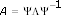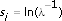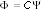# Building Stochastic State-Space Models (Advanced Signal Processing Toolkit)

LabVIEW 2014 Advanced Signal Processing Toolkit Help

Edition Date: June 2014

Part Number: 372656C-01

»View Product InfoDownload Help (Windows Only)

A stochastic state-space model describes an output-only dynamic system according to the following equations:

xk + 1 = Axk + wk

yk = Cxk + vk

 where yk is the (m × 1) multivariate time series with m variables xk is the state vector with n state variables n is the model order A is the state transition matrix with a dimension of n × n C is the measurement matrix, or state observation matrix, with a dimension of m × n wk and vk are the (n × 1) and (m × 1) noise vectors with a mean value of zero, respectively

The matrix A is defined as follows:where Λ is a diagonal matrix containing the complex eigenvalues λi(i = 1, 2, ..., n) of matrix A. Ψ contains the eigenvectors as columns.

You can obtain the modal pole si from the complex eigenvalues λi as follows:The eigenvalues of the state transition matrix A characterize the dynamic behavior of a physical system. By computing the state transition matrix A and measurement matrix C, you also can obtain the following modal parameters defined in the modal parametric model: natural frequencies, damping factors, resonance magnitudes, and resonance phases.

You can obtain the complex magnitude Gi vector for each modal pole si from the measurement matrix C, using the state observation equation as follows:Each column of the matrix Φ is associated with one complex magnitude Gi.

Use the TSA Stochastic State-Space Modeling VI to build stochastic state-space models for multivariate time series.